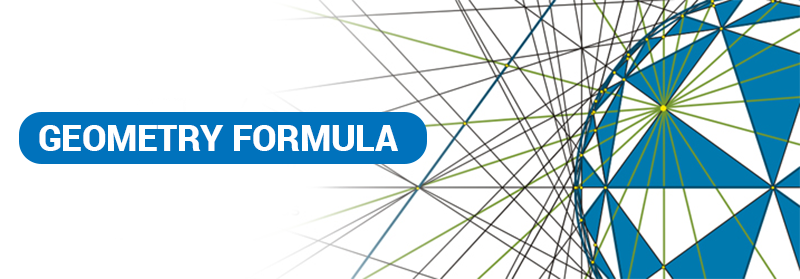# GMAT Quant: Geometry FormulaFor any Maths and Quantitative Aptitude sum, formulas are those fundamentals which help you to resonate and solve it. In GMAT Quant, this cheat-sheet list of formulas for Geometry, will assist you in brushing up your basics.

Perimeter Formulas

 Shape Formula Where, P = Perimeter and Square $P = 4s$ s = Sides Rectangle $P = 2l + 2w$ l  = Length w = Width Parallelogram $P = 2l + 2w$ l  = Length w = Width Trapezoid or Trapezium $P = s_{1} + s_{2} + b_{1} + b_{2}$ $s_{1}$ and $s_{2}$ are two sides and $b_{1}$ and $b_{2}$ are the two bases of the figure. Triangle $P = s_{1} + s_{2} + b$ $s_{1}$ and $s_{2}$ are two sides and b = base Rhombus $P = 4l$ l  = Length

## Area Formulas

 Shape Formula Where A = Area and Triangle $A = \frac{1}{2} \times b \times h$ b = base h = Vertical height Square $A = a^{2}$ a = length of side Rectangle $A = w \times h$ w = Width h = Height Parallelogram $A = b \times h$ b = base h = vertical height Trapezoid or Trapezium $A = \frac{1}{2} (a + b) \times h$ a, b = Length if two sides of the figure h = Vertical height Circle $A = \pi r^{2}$ r = Radius Ellipse $A = \pi ab$ a, b = Longest and Shortest Radius of the figure Sector of a Circle $A = \frac{1}{2}r^{2}\theta$ r = Radius $\theta$ = angle in radians Regular n-polygon $A = \frac{1}{4} \times n \times a^{2} \times \cot \frac{\pi}{n}$ n = Number of Sides a = Length of the sides

## Surface Area Formulas

 Shape Formula Where S = Surface Area and Rectangular Solid $S = 2lh + 2wh + 2wl$ l  = Length h = Heightw = Width Cube $S = 6s^{2}$ s = Sides of the Cube Right Circular Cylinder $S = 2\pi \times rh + 2\pi \times r^{2}$ h = Vertical Height of the Cylinder r  = Radius of the base Sphere $S = 4\pi \times r^{2}$ r  = Radius Right Circular Cone $S = \pi \times r \sqrt{r^{2} + h^{2}}$ h = Vertical height r  = Radius of the base Torus $S = \pi^{2} \times (R^{2} – r^{2})$ R = Radius of the larger base r = Radius of the smaller base

## Volume Formulas

 Shape Formula Where V = Volume and Rectangular Solid $V = lwh$ l  = Length h = Heightw = Width Cube $V = s^{3}$ s = Sides of the Cube Right Circular Cylinder $V = \pi \times r^{2}h$ h = Vertical Height of the Cylinder r  = Radius of the base Sphere $V = \frac{4}{3} r^{3}$ r  = Radius Right Circular Cone $V = \frac{1}{3} \pi r^{2}h$ h = Vertical height r  = Radius of the base Square or Rectangular Pyramid $V = \frac{1}{3} lwh$ l  = Length of the Base h = Vertical heightw = Width of the Base (In Case of Square, bothe l and w will be equal)

## Circle

Circumference of a Circle

$Circumference \; (C) = \pi d = 2 \pi r$

Where, d = diameter and r = Radius

Area of a Circle

$Area \; (A) = \pi r^{2}$$a^{2} + b^{2} = c^{2}$